PRIMARY

2 - 6

SECONDARY

7 - 10

SENIOR

11 - 12

Home

# Shapes, shape names and list of shapes

Theory & Practice Questions.

## Theory

### [Definition]

#### Properties

##### Parallelogram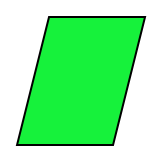Opposite sides are equal and parallel.
Opposite angles are equal.

##### Rhombus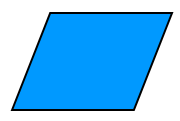Opposite sides are parallel.
All sides are equal.

##### Rectangle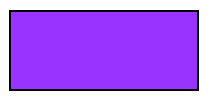Opposite sides are parallel and equal.
Each angle is a right angle.

##### Square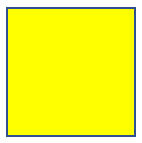Opposite sides are parallel.
All sides are equal.
Each angle is a right angle.

##### Kite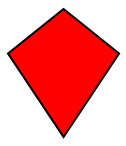Each two pairs of consecutive sides are equal.

##### Trapezium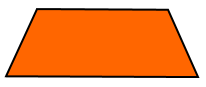Only one pair of opposite sides are parallel.

## Example

### [Investigation]

#### Identify, compare and describe quadrilaterals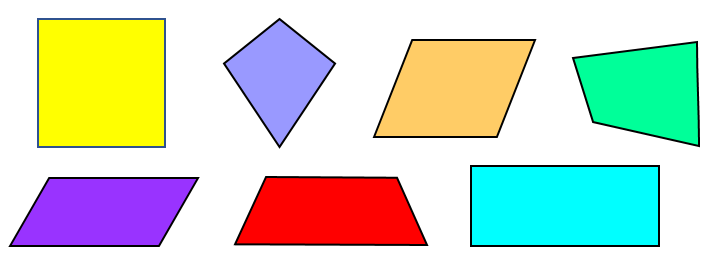Can you name all of these quadrilaterals?

Which of these have equal opposite sides?

Which of these have equal opposite angles?

Which of these have right angles?

## Question

Identify, compare and describe the following quadrilaterals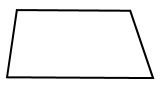• The shape on the left:
• The shape on the right:

## Explanation

The shape on the left is a kite, it has 2 pairs of equal and adjacent sides, and a pair of equal obtuse angles, and 2 acute angles.

The shape on the right is a trapezium, it has 1 pair of parallel lines, 2 obtuse angles and 2 acute angles.